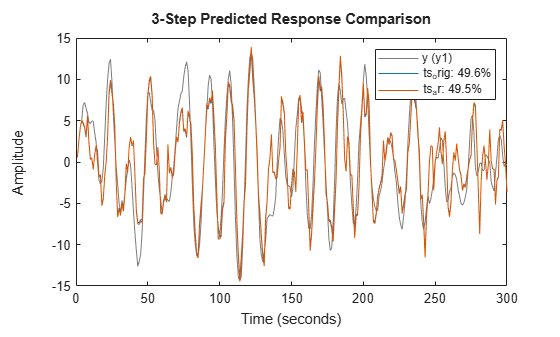Main Content

# Identify Time Series Models at the Command Line

This example shows how to simulate a time series and use parametric and nonparametric methods to estimate and compare time series models.

### Generate Model and Simulate Model Output

Generate time series data by creating and simulating an autoregressive (AR) polynomial model `ts_orig` of the form ${\mathit{y}}_{\mathit{k}}={\mathit{a}}_{1}\text{\hspace{0.17em}}{\mathit{y}}_{\mathit{k}-1}+{\mathit{a}}_{2}{\text{\hspace{0.17em}}\mathit{y}}_{\mathit{k}-2}+{\mathit{e}}_{\mathit{k}}$, where ${\mathit{e}}_{\mathit{k}}$ is random Gaussian noise. This noise represents an unmeasured input to the model. Since the model is a time series, there are no measured inputs.

Before calculating ${\mathit{e}}_{\mathit{k}}$, initialize the random number generator seed so that the noise values are repeatable.

```ts_orig = idpoly([1 -1.75 0.9]); rng('default') e = idinput(300,'rgs');```

Simulate the observed output `y_obs` of this model and convert `y_obs` to an `iddata` object `y` with the default sample time of one second. Plot the model output together with the input noise.

```y_obs = sim(ts_orig,e); y = iddata(y_obs); plot(e) hold on plot(y_obs) title('Input Noise and Original Model Output') legend('RGS Noise','Model Output') hold off```### Estimate Model and Compare Spectra

The functions `etfe` and `spa` provide two nonparametric techniques for performing spectral analysis. Compare the estimated power spectra from `etfe` and `spa` to the original model.

```ts_etfe = etfe(y); ts_spa = spa(y); spectrum(ts_etfe,ts_spa,ts_orig); legend('ts_{etfe}','ts_{spa}','ts_{orig}')```Now estimate a parametric model using the AR structure. Estimate a second-order AR model and compare its spectrum with the original model and the `spa` estimate.

```ts_ar = ar(y,2); spectrum(ts_spa,ts_ar,ts_orig); legend('ts_{spa}', 'ts_{ar}', 'ts_{orig}')```The AR model spectrum fits the original model spectrum more closely than the nonparametric models.

### Estimate and Compare Covariance

Calculate the covariance function for the original model and the AR model by convolving each model output with itself.

```ir_orig = sim(ts_orig,[1;zeros(24,1)]); Ry_orig = conv(ir_orig,ir_orig(25:-1:1)); ir_ar = sim(ts_ar,[1;zeros(24,1)]); Ry_ar = conv(ir_ar,ir_ar(25:-1:1));```

Also estimate the covariance `Ry` directly from the observed outputs `y` using `xcorr`.

`Ry = xcorr(y.y,24,'biased');`

Plot and compare the original and estimated covariances.

```plot(-24:24'*ones(1,3),[Ry_orig,Ry_ar,Ry]); legend('Ry_{orig}', 'Ry_{ar}', 'Ry')```The covariance of the estimated AR model, `Ry_ar`, is closer to the original covariance `Ry_orig`.

### Predict and Compare Model Outputs

Compare the three-step prediction accuracy, or fit percentage, for the original model and the AR model, using the function `compare`. Here, `compare` computes the predicted response of the `ts_orig` and `ts_ar` models with the original model output data `y`, assuming unmeasured input ${\mathit{e}}_{\mathit{k}}$ is zero. The fourth argument, `3`, is the number of steps to predict.

`compare(y,ts_orig,ts_ar,3);`The percentages in the legend are the fit percentages, which represent goodness of fit. The prediction accuracy is far from 100% even for the original model because the unmeasured model input ${\mathit{e}}_{\mathit{k}}$ is not accounted for in the prediction process. The fit value for the estimated AR model is close to the original model, indicating that the AR model is a good estimate.

## SupportGet trial now